$$\newcommand{\id}{\mathrm{id}}$$ $$\newcommand{\Span}{\mathrm{span}}$$ $$\newcommand{\kernel}{\mathrm{null}\,}$$ $$\newcommand{\range}{\mathrm{range}\,}$$ $$\newcommand{\RealPart}{\mathrm{Re}}$$ $$\newcommand{\ImaginaryPart}{\mathrm{Im}}$$ $$\newcommand{\Argument}{\mathrm{Arg}}$$ $$\newcommand{\norm}{\| #1 \|}$$ $$\newcommand{\inner}{\langle #1, #2 \rangle}$$ $$\newcommand{\Span}{\mathrm{span}}$$

2.5: Prime Factorization and the Least Common Multiple (Part 1)

•• Contributed by OpenStax
• Mathematics at OpenStax CNX

$$\newcommand{\vecs}{\overset { \rightharpoonup} {\mathbf{#1}} }$$

$$\newcommand{\vecd}{\overset{-\!-\!\rightharpoonup}{\vphantom{a}\smash {#1}}}$$

Skills to Develop

• Find the prime factorization of a composite number
• Find the least common multiple (LCM) of two numbers

be prepared!

Before you get started, take this readiness quiz.

1. Is $$810$$ divisible by $$2, 3, 5, 6,$$ or $$10$$? If you missed this problem, review Example 2.4.5.
2. Is $$127$$ prime or composite? If you missed this problem, review Example 2.4.8.
3. Write $$2 • 2 • 2 • 2$$ in exponential notation. If you missed this problem, review Example 2.1.5.

Find the Prime Factorization of a Composite Number

In the previous section, we found the factors of a number. Prime numbers have only two factors, the number $$1$$ and the prime number itself. Composite numbers have more than two factors, and every composite number can be written as a unique product of primes. This is called the prime factorization of a number. When we write the prime factorization of a number, we are rewriting the number as a product of primes. Finding the prime factorization of a composite number will help you later in this course.

Definition: Prime Factorization

The prime factorization of a number is the product of prime numbers that equals the number.

You may want to refer to the following list of prime numbers less than $$50$$ as you work through this section.

$$2, 3, 5, 7, 11, 13, 17, 19, 23, 29, 31, 37, 41, 43, 47$$

Prime Factorization Using the Factor Tree Method

One way to find the prime factorization of a number is to make a factor tree. We start by writing the number, and then writing it as the product of two factors. We write the factors below the number and connect them to the number with a small line segment—a “branch” of the factor tree.

If a factor is prime, we circle it (like a bud on a tree), and do not factor that “branch” any further. If a factor is not prime, we repeat this process, writing it as the product of two factors and adding new branches to the tree.

We continue until all the branches end with a prime. When the factor tree is complete, the circled primes give us the prime factorization.

For example, let’s find the prime factorization of $$36$$. We can start with any factor pair such as $$3$$ and $$12$$. We write $$3$$ and $$12$$ below $$36$$ with branches connecting them.The factor $$3$$ is prime, so we circle it. The factor $$12$$ is composite, so we need to find its factors. Let’s use $$3$$ and $$4$$. We write these factors on the tree under the $$12$$.The factor $$3$$ is prime, so we circle it. The factor $$4$$ is composite, and it factors into $$2 • 2$$. We write these factors under the $$4$$. Since $$2$$ is prime, we circle both $$2s$$.The prime factorization is the product of the circled primes. We generally write the prime factorization in order from least to greatest.

$$2 \cdot 2 \cdot 3 \cdot 3$$

In cases like this, where some of the prime factors are repeated, we can write prime factorization in exponential form.

$$2 \cdot 2 \cdot 3 \cdot 3$$

$$2^{2} \cdot 3^{2}$$

Note that we could have started our factor tree with any factor pair of $$36$$. We chose $$12$$ and $$3$$, but the same result would have been the same if we had started with $$2$$ and $$18$$, $$4$$ and $$9$$, or $$6$$ and $$6$$.

HOW TO: FIND THE PRIME FACTORIZATION OF A COMPOSITE NUMBER USING THE TREE METHOD

• Step 1. Find any factor pair of the given number, and use these numbers to create two branches.
• Step 2. If a factor is prime, that branch is complete. Circle the prime.
• Step 3. If a factor is not prime, write it as the product of a factor pair and continue the process.
• Step 4. Write the composite number as the product of all the circled primes.

Example $$\PageIndex{1}$$: prime factors

Find the prime factorization of $$48$$ using the factor tree method.

Solution

 We can start our tree using any factor pair of 48. Let's use 2 and 24. We circle the 2 because it is prime and so that branch is complete.Now we will factor 24. Let's use 4 and 6.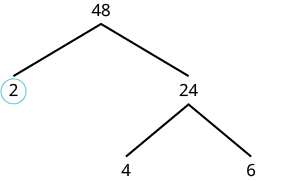Neither factor is prime, so we do not circle either. We factor the 4, using 2 and 2. We factor 6, using 2 and 3. We circle the 2s and the 3 since they are prime. Now all of the branches end in a prime.Write the product of the circled numbers. 2 • 2 • 2 • 2 • 3 Write in exponential form. 24 • 3

Check this on your own by multiplying all the factors together. The result should be $$48$$.

Exercise $$\PageIndex{1}$$

Find the prime factorization using the factor tree method: $$80$$

$$2 \cdot 2 \cdot 2 \cdot 2 \cdot 5, \text { or } 2^4 \cdot 5$$

Exercise $$\PageIndex{2}$$

Find the prime factorization using the factor tree method: $$60$$

$$2 \cdot 2 \cdot 3 \cdot 5, \text { or } 2^2 \cdot 3 \cdot 5$$

Example $$\PageIndex{2}$$: prime factors

Find the prime factorization of $$84$$ using the factor tree method.

Solution

 We start with the factor pair 4 and 21. Neither factor is prime so we factor them further.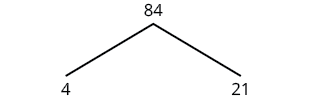Now the factors are all prime, so we circle them.Then we write 84 as the product of all circled primes. 2 • 2 • 3 • 7 = 22 • 3 • 7

Draw a factor tree of $$84$$.

Exercise $$\PageIndex{3}$$

Find the prime factorization using the factor tree method: $$126$$

$$2 \cdot 3 \cdot 3 \cdot 7, \text { or } 2 \cdot 3^2 \cdot 7$$

Exercise $$\PageIndex{4}$$

Find the prime factorization using the factor tree method: $$294$$

$$2 \cdot 3 \cdot 7 \cdot 7, \text { or } 2 \cdot 3 \cdot 7^2$$

Prime Factorization Using the Ladder Method

The ladder method is another way to find the prime factors of a composite number. It leads to the same result as the factor tree method. Some people prefer the ladder method to the factor tree method, and vice versa.

To begin building the “ladder,” divide the given number by its smallest prime factor. For example, to start the ladder for $$36$$, we divide $$36$$ by $$2$$, the smallest prime factor of $$36$$.To add a “step” to the ladder, we continue dividing by the same prime until it no longer divides evenly.Then we divide by the next prime; so we divide $$9$$ by $$3$$.We continue dividing up the ladder in this way until the quotient is prime. Since the quotient, $$3$$, is prime, we stop here. Do you see why the ladder method is sometimes called stacked division?

The prime factorization is the product of all the primes on the sides and top of the ladder.

$$2 \cdot 2 \cdot 3 \cdot 3$$

$$2^{2} \cdot 3^{2}$$

Notice that the result is the same as we obtained with the factor tree method.

HOW TO: FIND THE PRIME FACTORIZATION OF A COMPOSITE NUMBER USING THE LADDER METHOD

Step 1. Divide the number by the smallest prime.

Step 2. Continue dividing by that prime until it no longer divides evenly.

Step 3. Divide by the next prime until it no longer divides evenly.

Step 4. Continue until the quotient is a prime.

Step 5. Write the composite number as the product of all the primes on the sides and top of the ladder.

Example $$\PageIndex{3}$$: prime factors

Find the prime factorization of $$120$$ using the ladder method.

Solution

 Divide the number by the smallest prime, which is 2.Continue dividing by 2 until it no longer divides evenly.Divide by the next prime, 3.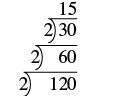The quotient, 5, is prime, so the ladder is complete. Write the prime factorization of 120. 2 • 2 • 2 • 3 • 5 23 • 3 • 5

Check this yourself by multiplying the factors. The result should be $$120$$.

Exercise $$\PageIndex{5}$$

Find the prime factorization using the ladder method: $$80$$

$$2 \cdot 2 \cdot 2 \cdot 2 \cdot 5, \text { or } 2^4 \cdot 5$$

Exercise $$\PageIndex{6}$$

Find the prime factorization using the ladder method: $$60$$

$$2 \cdot 2 \cdot 3 \cdot 5, \text { or } 2^2 \cdot 3 \cdot 5$$

Example $$\PageIndex{4}$$: prime factors

Find the prime factorization of $$48$$ using the ladder method.

Solution

 Divide the number by the smallest prime, 2.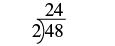Continue dividing by 2 until it no longer divides evenly.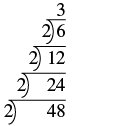The quotient, 3, is prime, so the ladder is complete. Write the prime factorization of 48. $$2 • 2 • 2 • 2 • 3$$ $$2^4 • 3$$

Exercise $$\PageIndex{7}$$

Find the prime factorization using the ladder method: $$126$$

$$2 \cdot 3 \cdot 3 \cdot 7, \text { or } 2 \cdot 3^2 \cdot 7$$

Exercise $$\PageIndex{8}$$

Find the prime factorization using the ladder method: $$294$$

$$2 \cdot 3 \cdot 7 \cdot 7, \text { or } 2 \cdot 3 \cdot 7^2$$